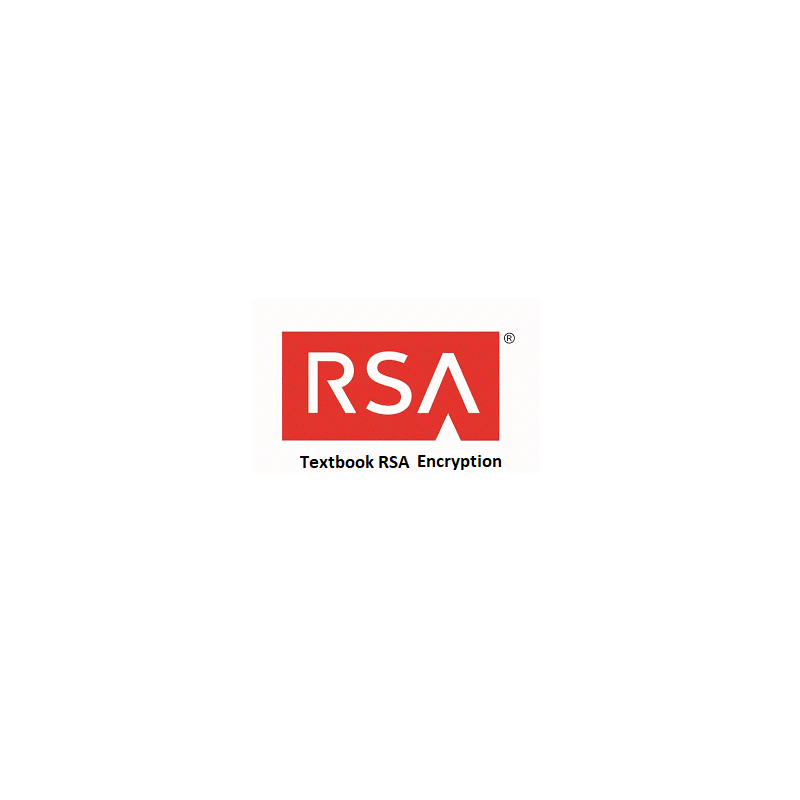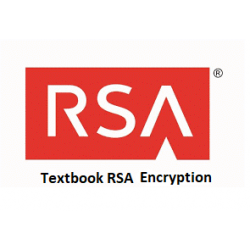•# RSA Textbook Encryption

€2.00
Tax included

RSA textbook encryption.

Quantity

COMPLETED.

Task for the Student is to generate RSA system parameters and send generated Public Key to the Mentor. Student must write current day ant time in the format DDhhmm encrypt it and send ciphertext to the Mentor.

After Student receives Mentor’s Public Key and ciphertext of unknown message, he must decrypt it and find plaintext of this ciphertext. He must encrypt this plaintext and send it for decryption to the Mentor.

Parameter values sent by Alice are included in brackets [ ] and must be entered in the corresponding input fields

The following functions are used in the protocol:

>> genprime(14)

>> gcd(a,b)

>> mod(a,n)

>> eeuklid(e,ϕ)

>>mod_exp(a,x,n)

1. Compute RSA module n=pq by generating two prime numbers of 14 bit length using function

>> genprime(14)

Send numbers [p, q] for verification to the Mentor.

1. If it is OK, find Euler’s totient function’s ϕ(n) value ϕ. Notice, that ϕ can be computed very effectively when p and q are given.

Send [ϕ] for verification to the Mentor.

1. If it is OK, choose RSA exponent e satisfying condition        gcd(e, ϕ)=1 and send computed RSA Public Key [n, e] for verification to the Mentor.

1. If it is OK, find RSA private key d using extended Euclidean algorithm

>> eeuklid(e,ϕ)

Send [d] for verification to the Mentor.

1. Mentor sends You his Public Key is nM=….. , eM=….. together with the ciphertext CM=….. corresponding to encrypted random number R. Find this random number by decrypting CM, encrypt R by finding ciphertext CR and send [CR] for decryption to the Mentor.

3098 Items